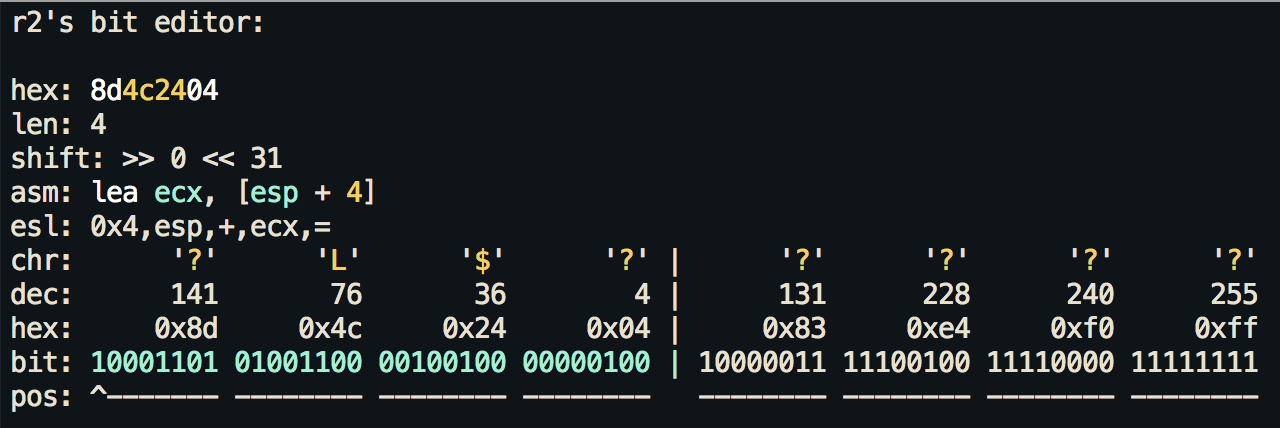# `d[f?]` define function, data, code, ..

• `d``\$` define flag size
• `d``1` edit bits
• Screenshot• `d``b` set as byte B set as short word (2 bytes) c set as code C define flag color (fc)

• `d``d` set as data
• `d``e` end of function
• Visual mode `de` Set end of function

• `d``f` analyze function
• Visual mode `df` anlyze the next function

• `d``F` format
• `d``i` immediate base (b(in), o(ct), d(ec), h(ex), s(tr)) j merge down (join this and next functions)
• `d``k` merge up (join this and previous function)
• `d``h` define anal hint
• `d``m` manpage for current call
• `d``n` rename flag used at cursor
• `d``r` rename function
• `d``R` find references /r
• `d``s` set string
• `d``S` set strings in current block
• `d``u` undefine metadata here
• `d``x` find xrefs to current address (./r)
• `d``w` set as 32bit word
• `d``W` set as 64bit word
• `d``q` quit menu
• `d``z` zone flag